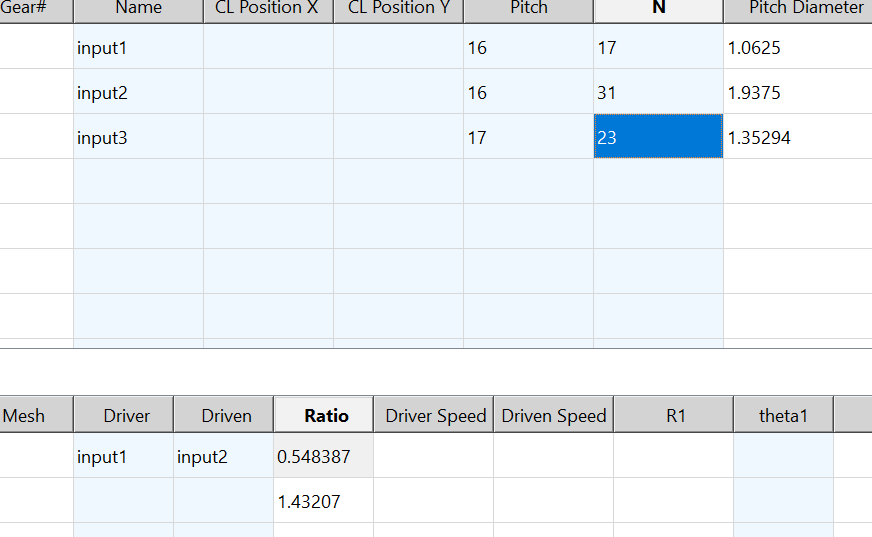# Qtableview calculation in column

•i have two tables(qtableview)
so how can i store the column data so i can use everywhere as now done like this

``````for (int i = 0, maxI = table1->rowCount();i <= maxI;++i) {
bool ok1, ok2;
double n = table1->data(table1->index(i, 5)).toDouble(&ok1);
double pitch = table1->data(table1->index(i, 4)).toDouble(&ok2);
if (ok1 && ok2 && pitch && n != 0)
table1->setData(table1->index(i, 8), (n+2)/pitch);
}
how can i store this setdata in some variable so can use in other column calculations ?
``````

also in table2 col 1and 2 combobox based on user input in table1 col1
so now when user select in table2 as col1 as input 1 col2 input 2 if user select input 2 input 3 so that pitchdiameter ratio of these should result in table2 col3 how to do this?

• also you can in table2 col 1and 2 combobox based on user input in table1 col1
so now when user select in table2 as col1 as input 1 col2 input 2 if user select input 2 input 3 so that pitchdiameter ratio of these should result in table2 col3 how to do this?

Maybe someone can understand what you are saying here, but I can't.

how can i store this setdata in some variable so can use in other column calculations ?

You just stored it in `table1->index(i, 8)`. So you can retrieve it from `table1->index(i, 8).data()`.

[BTW: `i <= maxI`: shouldn't this be `i < maxI`?]

• in table2 column 1&2 are combobox delegate and values are from table1 column1
so when user select in table2 column 1 &2 -input1 input2 or input2/3 so in table2 column 3 there will division of column6 table1(pitchdiameter) value (row 1 /row 2 value)
eg. if in table2 user select input1 input 3 in column1 &2 so now in column 3 result (ratio )is table1 col6 (row 1 /row 3 value)
if in table2 user select input2 input 3 in column1 &2 so now in column 3 result (ratio )is table1 col6 (row 2/row 3 value)
if in table2 user select input1 input 2 in column1 &2 so now in column 3 result (ratio )is table1 col6 (row 1/row 2 value)

• any suggestions how to do this ??

• @n-2204
No, because at least for myself I find your explanation of what you are trying to achieve indecypherably complex! If you want help, can't you rephrase your question to be minimal and much simpler. Unless someone else understands....

• how can i get selection value of combobox delegate from qtableview?